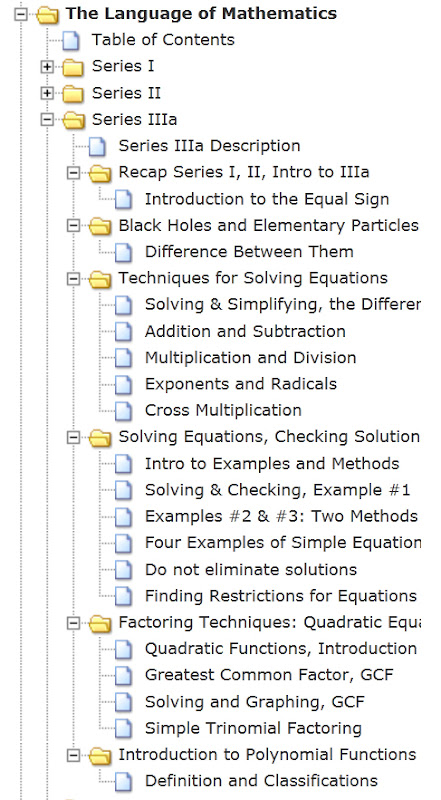| | | | |

## Tuesday, March 6, 2012

### Series IIIa Description: What's Contained in this Series

In this series we learn different techniques for solving equations, combine and isolate variables, talk about the equal sign, how to move around an equation, discuss the graphical meaning for solving equations, learn about restrictions, and how we would go about checking solutions.

Also included is an introduction to polynomial equations and functions, a discussion of their classifications, and factoring techniques, beginning with the Greatest Common Factor (GCF) and Simple Trinomial Factoring (Factoring techniques for polynomials is continued in Series IIIb).

In addition to the above, we also take a brief look at the difference between black holes and elementary particles.

Full list and links for all the videos contained in the series available at: Table of Contents: The Language of Mathematics - Series IIIa.

See Videos Available for Download for information on the torrent for this series.

For ease of reference, included below is the expanded image of the tree menu provided in the left column of this site. Specific topics can be found through the Index (left column).

Section 1: Summary of Series I and II, and an Introduction to Series IIIa
Section 2: Black Holes and Elementary Particles
Section 3: Techniques for Solving Equations
Section 4: Solving Equations and Checking Solutions, Examples and Methods
Section 5: Solving Quadratic Equations: Factoring Techniques
Section 6: Introduction to Polynomial FunctionsFrom Series IIIa Tree Menu - The Language of Mathematics
1.